Piecewise Defined Functions (or Split Definition Functions)

Example 1:    Graph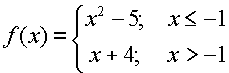• Open a Graph & Geometry Page
• Move to the command line to enter the function.
• Pressto get the template for piecewise entry. Choose the 7th template from the left, as it is designed for entering two "pieces". If you have more than two "pieces", choose the 8th template from the left.
Press ENTER.
• Use TAB to move within the template.
• Remember that CTRL < will give the symbol for "less than or equal to".

(or CTRL - T). (press CTRL - Z to remove table)
 NOTE:  The calculator will not draw the "open" or "closed" circles at the endpoints of sections of the graph.  You will have to draw the appropriate circles when copying the graph. "open circle"  < or > "closed circle" < or >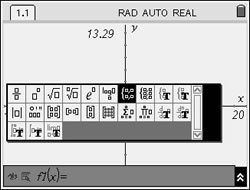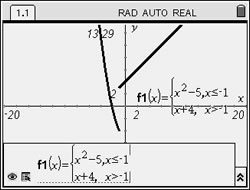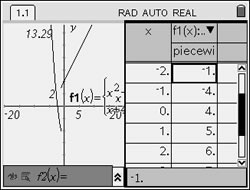Example 2:    Graph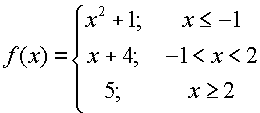• Open a Graph & Geometry Page
• Move to the command line to enter the function.
• Pressto get the template for piecewise entry. Choose the 8th template from the left. This template will allow you to control how many "pieces" of the function are entered.
Press ENTER. Press ENTER.

• Use TAB to move within the template.
• Remember that CTRL < will give the symbol for "less than or equal to".
 NOTE:  The calculator will not draw the "open" or "closed" circles at the endpoints of sections of the graph.  You will have to draw the appropriate circles when copying the graph. "open circle"  < or > "closed circle" < or >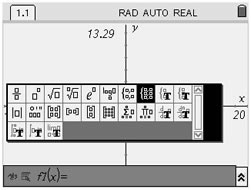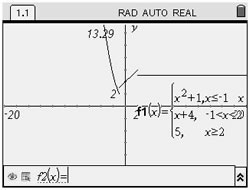NOTE: The TI-Nspire has NO problem with entering -1 < x < 2. Many computers and calculators cannot interpret expressions such as -1 < x < 2 as we do in mathematics.  These computers and calculators see this expression as ONLY -1 < x (and they ignore the second part of the condition).  To force them to see the second condition, it it necessary to write the expression -1 < x < 2 as (-1 < x) and (x < 2). This is NOT the case with the Nspire. This calculator understands the complete meaning of -1 < x < 2.